# Top Must Know Machine Learning Algorithms In 2023

Machine learning can be defined as a learning task where a function is obtained that maps an input to an output model based on input and output pairs. In this method, a pair of input objects and an output object are analyzed by a supervised learning algorithm to produce the much-needed derived function.

## Machine learning algorithms

### Linear regression

The process of linear regression, the relationship between the independent and dependent variables is established by fitting them on a straight line. This line is called the regression line and is represented. The coefficients A&B are derived by minimizing the sum of squares of the difference in distance between the data points and the regression line.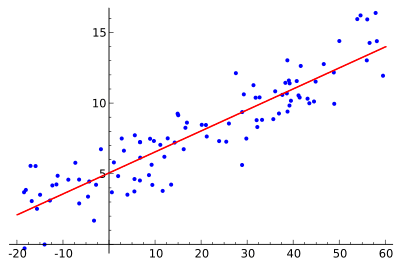### Logistic regression

Logistic regression is used to estimate discrete values ​​from a set of independent variables. It helps predict the probability of an event by fitting the data to a logit function. Methods such as interaction terms, feature elimination, regularization techniques, and the use of a non-linear model can help improve logistic regression models.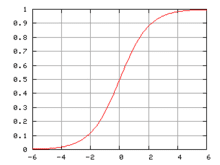### Decision tree

A decision tree in machine learning algorithms, which is one of the very popular ones today. It is a supervised learning algorithm used for problem classification. Works well with both continuous and categorical dependent variables.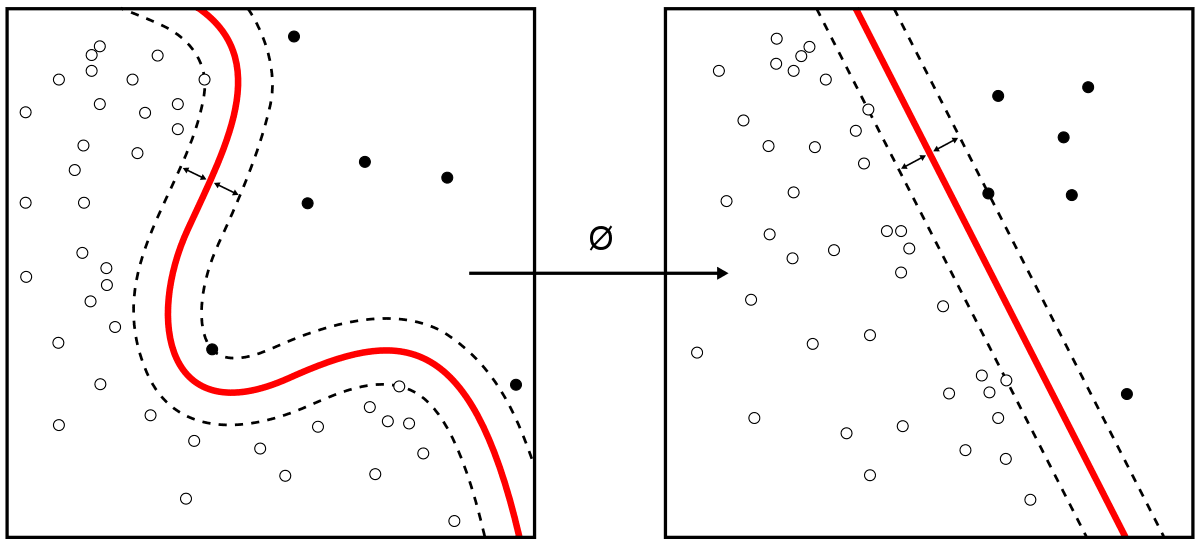### SVM

SVM is one of the machine learning algorithms used to classify algorithms in which you plot the raw data as points in an n-dimensional space. In this algorithm, we divide the population into two or more homogeneous sets based on the most significant attributes of the independent variables.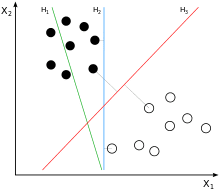### Naive bayes algorithm

A naive Bayes classifier assumes that the presence of a certain feature in a class is unrelated to the presence of any other feature. Although these features are related, a Naive Bayes classifier would consider all of these features independently when calculating the probability of a particular outcome.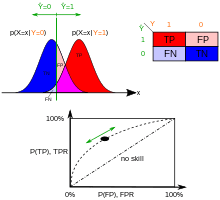### KNN

These algorithms can be applied to both regression problems and classification. It is one of the machine learning algorithms widely used to solve classification problems.

### K-Means

Data are classified into a certain number of clusters in such a way that the data points within the cluster are homogeneous and heterogeneous from the data in other clusters.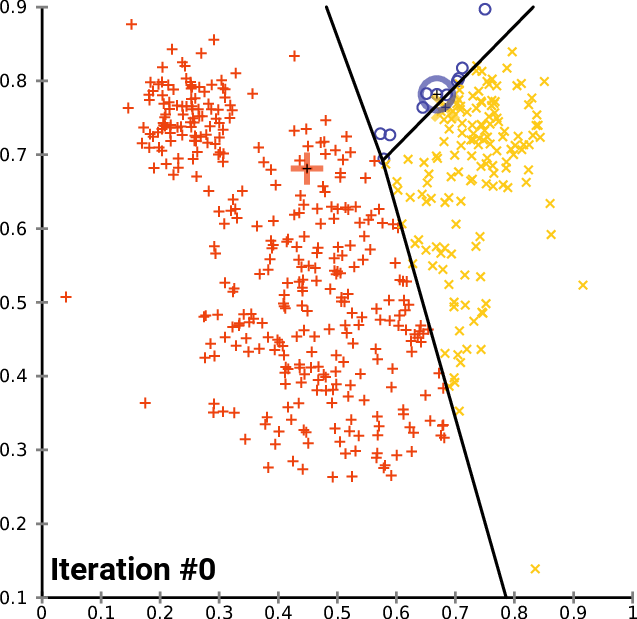### Self-organizing maps

Data visualization attempts to solve the problem that humans cannot easily visualize high-dimensional data. SOMs are created to help users understand this high-dimensional information.

### Deep belief networks

DBNs are generative models that consist of multiple layers of stochastic, latent variables. Latent variables have binary values ​​and are often called latent units. DBNs are a stack of Boltzmann machines with interconnections between layers, and each RBM layer communicates with the previous and subsequent layers.

### Automatic encoders

Autoencoders are a specific type of feedforward neural network in which the input and output are identical. They are trained neural networks that replicate data from the input layer to the output layer. Autoencoders are used for purposes such as pharmaceutical discovery, popularity prediction, and image processing.

## Conclusion

This program will provide you with deep knowledge of Python, tensor flow deep learning algorithm, natural language processing, speech recognition, computer vision and reinforcement learning.